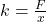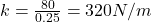## A mass on a horizontal surface is connected to the spring and pulled to the right along the surface stretching the spring by 25 cm. if the p

Question

A mass on a horizontal surface is connected to the spring and pulled to the right along the surface stretching the spring by 25 cm. if the pulling force exerted on the mass was 80.N, determine the spring constant of the spring.

in progress 0
2 months 2021-07-25T05:30:34+00:00 1 Answers 2 views 0

320 N/m

Explanation:

From Hooke’s law, we deduce that

F=kx where F is applied force, k is spring constant and x is extension or compression of spring

Making k the subject of formula thenConversion

1m equals to 100cm

Xm equals 25 cm

25/100=0.25 m

Substituting 80 N for F and 0.25m for x thenTherefore, the spring constant is equal to 320 N/m